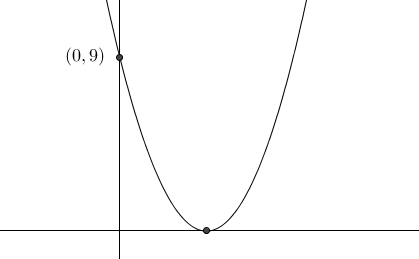Graph and discriminant

Algebra Level 2If the curve $y=x^2+bx+c$ touches the $x$-axis at some point and intersects the y-axis at $(0,9)$, find the absolute value of $b$.

×